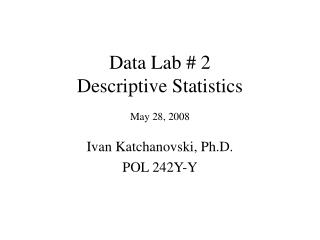# Data Lab # 2 Descriptive Statistics May 28, 2008 - PowerPoint PPT PresentationDownload PresentationData Lab # 2 Descriptive Statistics May 28, 2008

Data Lab # 2 Descriptive Statistics May 28, 2008Download Presentation## Data Lab # 2 Descriptive Statistics May 28, 2008

- - - - - - - - - - - - - - - - - - - - - - - - - - - E N D - - - - - - - - - - - - - - - - - - - - - - - - - - -
##### Presentation Transcript

1. Data Lab # 2Descriptive StatisticsMay 28, 2008 Ivan Katchanovski, Ph.D. POL 242Y-Y

2. Measures of Central Tendency: The Mode • The mode is the only measure of central tendency for nominal variables • The mode can also be found for ordinal and interval-ratio variables • Example: select a nominal variable • colony (World dataset) • SPSS Command: • Analyze-Descriptive Statistics-Frequencies-Statistics-Mode • Identify the mode by a value in statistics box • Identify the mode by a value with the highest frequency • Identify the mode by a value with the highest percentage in “valid percent” column

3. Measures of Central Tendency: The Median • The median is appropriate for ordinal and interval-ratio variables • The median is not appropriate for nominal variables • Example: Select an ordinal variable • Economic development (World dataset) • SPSS Command: • Analyze-Descriptive Statistics-Frequencies-Median • Identify the median by a value in statistics box • Identify the median by a value below which 50% of cases lie in “cumulative percent” column

4. Measures of Central Tendency: The Mean • The mean is only appropriate for interval-ratio variables • Example: Select interval ratio variable • GDP per capita \$1000 (World dataset) • SPSS Command: • Analyze-Descriptive Statistics-Frequencies-Statistics-Mean • Identify the mean by a value in statistics box

5. Graphic Description of Variables: Bar Chart • Bar chart • Most appropriate for nominal and ordinal variables • Shows dispersion of a variable • Example: select a nominal variable • colony (World dataset) • SPSS Commands: • Analyze-Descriptive Statistics-Frequencies-Charts-Bar charts-Percentages • Open Chart Editor: Select chart-Edit-SPSS Chart Object-Open • In Chart Editor: Options-Title • In Chart Editor: Elements-Show Data Labels

6. Graphic Description of Variables: Pie Chart • Pie chart • Most appropriate for nominal and ordinal variables with small number of values (categories) • Example: select an ordinal variable • economic development (World dataset) • SPSS Commands: • Analyze-Descriptive Statistics-Frequencies-Charts-Pie charts-Percentages • Open Chart Editor: Select chart-Edit-SPSS Chart Object-Open • In Chart Editor: Options-Title • In Chart Editor: Elements-Show Data Labels

7. Graphic Description of Variables: Histogram • Histogram • Most appropriate for interval-ratio variables with large number of values • Shows dispersion of a variable • Example: select an interval-ratio variable • GDP per capita \$1000 (World dataset) • SPSS Commands: • Analyze-Descriptive Statistics-Frequencies-Charts-Histograms • Open Chart Editor: Select chart-Edit-SPSS Chart Object-Open • In Chart Editor: Options-Title • In Chart Editor: Elements-Show Data Labels

8. Skewness of Distributions • Skewness of distributions • Appropriate for interval-ratio variables • Example: select an interval-ratio variable • GDP per capita \$1000 (World dataset) • SPSS Commands: • Analyze-Descriptive Statistics-Frequencies-Statistics-Skewness/Mean/Median • Unskewed distribution if skewness statistics=0 or mean=median • Positively skewed distribution if skewness statistics>0 or mean>median • Negatively skewed distribution if skewness statistics<0 or mean<median

9. Helpful SPSS Options • SPSS option for displaying variable labels instead of variable names • Open the 2000 World Values Survey Dataset • Edit-Options-General-Display labels • SPSS option for selecting cases • Example: selecting Canadian respondents from the World Values Survey dataset • Identify value for Canada (12) in “nation” variable (v2) • Data-Select cases-If: v2=12

10. Dependent and independent variables • Describing dependent and independent variables • The 2000 World Values Survey • Questionnaires • Country specific questionnaires online at http://www.worldvaluessurvey.org/ • “Documentation of the Values Surveys” & “1999-2004 wave” • Select Canadian questionnaire and Canadian sample of the 2000 World Values Survey dataset • Identify dependent variable • Identify independent variable • Produce appropriate descriptive statistics and charts